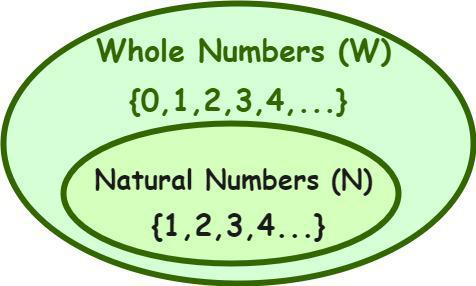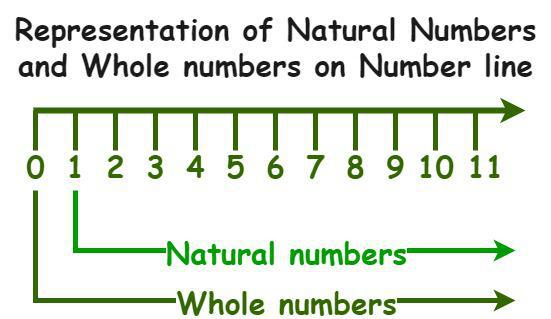GFG App
Open AppBrowser
Continue

# Properties of Whole Numbers

A number system refers to a system that represents numbers, where a number is defined as the mathematical value that helps to count, measure, or label and perform various mathematical calculations. We have various types of numbers based on their properties, such as natural numbers, whole numbers, integers, rational and irrational numbers, real numbers, etc. In a number system, these numbers are used as digits. A number system also helps us to perform various arithmetic operations such as addition, subtraction, and division. A binary number system, an octal number system, a decimal number system, and a decimal number system are different types of number systems.

## Whole Numbers

The set of all-natural numbers and zero is referred to as whole numbers. Whole numbers are a part of real numbers that include only positive numbers and zero, i.e., they do not include negative numbers, decimals, or fractions. In mathematics, whole numbers are denoted by the letter W. The set of whole numbers is given as W = {0, 1, 2, 3,…}, so we can say that whole numbers are a set of non-negative integers. Whole numbers are a set of counting numbers starting from 0, 1, 2, 3, 4, and so on. As whole numbers start from 0, 0 is the smallest whole number. On a number line, zero lies between the positive and negative numbers. We can also perform arithmetic operations such as addition, subtraction, multiplication, and division on whole numbers.## Properties of Whole Numbers

The fundamental arithmetic operations such as addition, subtraction, multiplication, and division on whole numbers lead to four main properties of whole numbers, such as closure property, commutative property, associative property, and distributive property.

### Closure property

The sum and product of two whole numbers is also a whole number and can be closed under addition and multiplication.

For example, the sum of two whole numbers, 14 and 18, is 32, which is also a whole number [14+18 = 32], and their product is 252, which is also a whole number [14 × 18 = 252].

### Commutative Property

The sum and product of whole numbers remain the same even if the order of the numbers is interchanged. Let us consider two whole numbers, ‘a’ and ‘b’. Then, according to the commutative property,

• a + b = b + a
• a × b = b × a

Note that the commutative property does not hold true in the case of subtraction and division of whole numbers.

When any whole number is added to 0, then its value remains unchanged. For example, let us consider a whole number “a”, then

• a + 0 = 0 + a = a

Example: 5 + 0 = 0 + 5 = 5

### Multiplicative Identity

When any whole number is multiplied by 1, then its value remains unchanged. For example, let us consider a whole number “a”, then

• a × 1 = 1 × a = a

Example: 8 × 1 = 1 × 8 = 8

### Associative property

The sum or product of any three whole numbers remains unchanged even if the grouping of numbers is changed. Let us consider three whole numbers, ‘a’, ‘b’, and ‘c’. Then, according to the associative property,

• a + (b + c) = (a + b) + c
• a × (b × c) = (a × b) × c

### Distributive Property

The distributive property states that the multiplication of a whole number is distributed over the sum or difference of the whole numbers. Let us consider three whole numbers, ‘a’, ‘b’, and ‘c’. Then the distributive property states that,

• a × (b + c) = (a × b) + (a × c)
• a × (b – c) = (a × b) – (a × c)

## Difference between Whole Numbers and Natural Numbers## Solved Examples on Whole Numbers

Example 1: Identify the whole numbers among the set of numbers { -5, -4.25, 0, 2/5, 8, 19, 68}.

Solution:

The set of all-natural numbers and zero is referred to as whole numbers. In mathematics, the set of whole numbers is given as W = {0, 1, 2, 3, 4,…}.

Hence, the whole numbers among the given numbers are {0, 8, 19, and 68}.

Example 2: Are -135, 51, 112, 169, and 1829 whole numbers?

Solution:

As -135 is a negative integer, all the given numbers except -135 are whole numbers, i.e., 51, 112, 169, and 1829 are whole numbers.

Example 3: Solve 14 × (3 + 7) using the distributive property.

Solution:

The distributive property of multiplication over the addition of whole numbers is:

a × (b + c) = (a × b) + (a × c)

So, 14 × (3 + 7) = (14 × 3) + (14 × 7)

= 42 + 98

= 140

Hence, the value of 14 × (3 + 7) is 140.

Example 4: When is the product of two whole numbers zero?

Solution:

The product of two whole numbers is zero when one of them is zero.

For example, the product of 0 and 6 is 0 (0 × 6 = 0) and the product of 13 and 0 is 0 (13 × 0 = 0).

The product of two whole numbers is zero when both of them are zero, i.e., 0 × 0 = 0.

So, the product of two whole numbers is zero when either of them is zero or both of them are zero.

Example 5: Find the value of 8 × (36 – 6), using the distributive property.

Solution:

The distributive property of multiplication over the subtraction of whole numbers is:

a × (b  – c) = (a × b) – (a × c)

So, 8 × (36 – 6) = (8 × 36) – (8 × 6)

= 288 – 48

= 240

Thus, the value of 8 × (36 – 6) is 240.

## FAQs on Whole Numbers

Question 1: What is meant by whole numbers in math?

In math, the set of all-natural numbers and zero is referred to as whole numbers. Whole numbers are a set of counting numbers starting from 0, 1, 2, 3, 4, and so on. Whole numbers are a part of real numbers that include only positive numbers and zero, i.e., they do not include negative numbers, decimals, or fractions.

Question 2: What is the symbol for the set whole numbers and write some examples of whole numbers.

In math, whole numbers are denoted by the letter W, and the set of whole numbers is given as W = {0, 1, 2, 3, 4,…}. Every value in the W = {0, 1, 2, 3, 4,…} set is an example of a whole number. Some examples of whole numbers are 0, 1, 2, 5, 10, 21, etc.

Question 3: Can whole numbers be negative? What is the smallest whole number?

In math, the set of all-natural numbers and zero is referred to as whole numbers. Whole numbers are a part of real numbers that include only positive numbers and zero, i.e., they do not include negative numbers, decimals, or fractions. The set of whole numbers is given as W = {0, 1, 2, 3, 4,…}. Thus, whole numbers cannot be negative. The smallest whole number is 0, as whole numbers start with 0.

Question 4: Which is the whole number that is not a natural number?